oomph::GMRES< MATRIX > Class Template Reference

//////////////////////////////////////////////////////////////////// //////////////////////////////////////////////////////////////////// More...

#include <iterative_linear_solver.h>Inheritance diagram for oomph::GMRES< MATRIX >:

## Public Member Functions

GMRES ()
Constructor. More...

virtual ~GMRES ()
Destructor (cleanup storage) More...

GMRES (const GMRES &)=delete
Broken copy constructor. More...

void operator= (const GMRES &)=delete
Broken assignment operator. More...

void disable_resolve ()
Overload disable resolve so that it cleans up memory too. More...

function to enable the computation of the gradient More...

void solve (Problem *const &problem_pt, DoubleVector &result)
Solver: Takes pointer to problem and returns the results vector which contains the solution of the linear system defined by the problem's fully assembled Jacobian and residual vector. More...

void solve (DoubleMatrixBase *const &matrix_pt, const DoubleVector &rhs, DoubleVector &solution)
Linear-algebra-type solver: Takes pointer to a matrix and rhs vector and returns the solution of the linear system. More...

void solve (DoubleMatrixBase *const &matrix_pt, const Vector< double > &rhs, Vector< double > &result)
Linear-algebra-type solver: Takes pointer to a matrix and rhs vector and returns the solution of the linear system Call the broken base-class version. If you want this, please implement it. More...

void resolve (const DoubleVector &rhs, DoubleVector &result)
Re-solve the system defined by the last assembled Jacobian and the rhs vector specified here. Solution is returned in the vector result. More...

unsigned iterations () const
Number of iterations taken. More...

bool iteration_restart () const
access function indicating whether restarted GMRES is used More...

void enable_iteration_restart (const unsigned &restart)
switches on iteration restarting and takes as an argument the number of iterations after which the construction of the orthogonalisation basis vectors should be restarted More...

void disable_iteration_restart ()
switches off iteration restart More...

void set_preconditioner_LHS ()
Set left preconditioning (the default) More...

void set_preconditioner_RHS ()
Enable right preconditioning. More...Public Member Functions inherited from oomph::IterativeLinearSolver
IterativeLinearSolver ()
Constructor: Set (default) trivial preconditioner and set defaults for tolerance and max. number of iterations. More...

IterativeLinearSolver (const IterativeLinearSolver &)=delete
Broken copy constructor. More...

void operator= (const IterativeLinearSolver &)=delete
Broken assignment operator. More...

virtual ~IterativeLinearSolver ()
Destructor (empty) More...

Preconditioner *& preconditioner_pt ()
Access function to preconditioner. More...

Preconditioner *const & preconditioner_pt () const
Access function to preconditioner (const version) More...

double & tolerance ()

unsigned & max_iter ()

void enable_doc_convergence_history ()
Enable documentation of the convergence history. More...

void disable_doc_convergence_history ()
Disable documentation of the convergence history. More...

void open_convergence_history_file_stream (const std::string &file_name, const std::string &zone_title="")
Write convergence history into file with specified filename (automatically switches on doc). Optional second argument is a string that can be used (as a zone title) to identify what case we're running (e.g. what combination of linear solver and preconditioner or parameter values are used). More...

void close_convergence_history_file_stream ()
Close convergence history output stream. More...

double jacobian_setup_time () const
returns the time taken to assemble the jacobian matrix and residual vector More...

double linear_solver_solution_time () const
return the time taken to solve the linear system More...

virtual double preconditioner_setup_time () const
returns the the time taken to setup the preconditioner More...

void enable_setup_preconditioner_before_solve ()
Setup the preconditioner before the solve. More...

void disable_setup_preconditioner_before_solve ()
Don't set up the preconditioner before the solve. More...

void enable_error_after_max_iter ()
Throw an error if we don't converge within max_iter. More...

void disable_error_after_max_iter ()
Don't throw an error if we don't converge within max_iter (default). More...

void enable_iterative_solver_as_preconditioner ()
Enables the iterative solver be used as preconditioner (when calling the solve method it bypass the setup solver method – currently only used by Trilinos solver —) More...

void disable_iterative_solver_as_preconditioner ()
Disables the iterative solver be used as preconditioner (when calling the solve method it bypass the setup solver method – currently only used by Trilinos solver —) More...Public Member Functions inherited from oomph::LinearSolver
LinearSolver ()
Empty constructor, initialise the member data. More...

LinearSolver (const LinearSolver &dummy)=delete
Broken copy constructor. More...

void operator= (const LinearSolver &)=delete
Broken assignment operator. More...

virtual ~LinearSolver ()
Empty virtual destructor. More...

void enable_doc_time ()
Enable documentation of solve times. More...

void disable_doc_time ()
Disable documentation of solve times. More...

bool is_doc_time_enabled () const
Is documentation of solve times enabled? More...

bool is_resolve_enabled () const
Boolean flag indicating if resolves are enabled. More...

virtual void enable_resolve ()
Enable resolve (i.e. store matrix and/or LU decomposition, say) Virtual so it can be overloaded to perform additional tasks. More...

virtual void solve_transpose (Problem *const &problem_pt, DoubleVector &result)
Solver: Takes pointer to problem and returns the results vector which contains the solution of the linear system defined by the problem's fully assembled Jacobian and residual vector (broken virtual). More...

virtual void solve_transpose (DoubleMatrixBase *const &matrix_pt, const DoubleVector &rhs, DoubleVector &result)
Linear-algebra-type solver: Takes pointer to a matrix and rhs vector and returns the solution of the linear system. More...

virtual void solve_transpose (DoubleMatrixBase *const &matrix_pt, const Vector< double > &rhs, Vector< double > &result)
Linear-algebra-type solver: Takes pointer to a matrix and rhs vector and returns the solution of the linear system. More...

virtual void resolve_transpose (const DoubleVector &rhs, DoubleVector &result)
Solver: Resolve the system defined by the last assembled jacobian and the rhs vector. Solution is returned in the vector result. (broken virtual) More...

function to disable the computation of the gradient required for the globally convergent Newton method More...

function to reset the size of the gradient before each Newton solve More...

function to access the gradient, provided it has been computed More...Public Member Functions inherited from oomph::DistributableLinearAlgebraObject
DistributableLinearAlgebraObject ()
Default constructor - create a distribution. More...

DistributableLinearAlgebraObject (const DistributableLinearAlgebraObject &matrix)=delete
Broken copy constructor. More...

void operator= (const DistributableLinearAlgebraObject &)=delete
Broken assignment operator. More...

virtual ~DistributableLinearAlgebraObject ()
Destructor. More...

unsigned nrow () const
access function to the number of global rows. More...

unsigned nrow_local () const
access function for the num of local rows on this processor. More...

unsigned nrow_local (const unsigned &p) const
access function for the num of local rows on this processor. More...

unsigned first_row () const
access function for the first row on this processor More...

unsigned first_row (const unsigned &p) const
access function for the first row on this processor More...

bool distributed () const
distribution is serial or distributed More...

bool distribution_built () const
if the communicator_pt is null then the distribution is not setup then false is returned, otherwise return true More...

void build_distribution (const LinearAlgebraDistribution *const dist_pt)
setup the distribution of this distributable linear algebra object More...

setup the distribution of this distributable linear algebra object More...

## Private Member Functions

void solve_helper (DoubleMatrixBase *const &matrix_pt, const DoubleVector &rhs, DoubleVector &solution)
General interface to solve function. More...

void clean_up_memory ()
Cleanup data that's stored for resolve (if any has been stored) More...

void update (const unsigned &k, const Vector< Vector< double > > &H, const Vector< double > &s, const Vector< DoubleVector > &v, DoubleVector &x)
Helper function to update the result vector using the result, x=x_0+V_m*y. More...

void generate_plane_rotation (double &dx, double &dy, double &cs, double &sn)
Helper function: Generate a plane rotation. This is done by finding the values of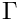(i.e. cs) and \sin(\theta) (i.e. sn) such that: More...

void apply_plane_rotation (double &dx, double &dy, double &cs, double &sn)
Helper function: Apply plane rotation. This is done using the update: More...

## Private Attributes

unsigned Iterations
Number of iterations taken. More...

unsigned Restart
The number of iterations before the iteration proceedure is restarted if iteration restart is used. More...

bool Iteration_restart
boolean indicating if iteration restarting is used More...

MATRIX * Matrix_pt
Pointer to matrix. More...

bool Resolving
Boolean flag to indicate if the solve is done in re-solve mode, bypassing setup of matrix and preconditioner. More...

bool Matrix_can_be_deleted
Boolean flag to indicate if the matrix pointed to be Matrix_pt can be deleted. More...

bool Preconditioner_LHS
boolean indicating use of left hand preconditioning (if true) or right hand preconditioning (if false) More...

double Preconditioner_application_time
Storage for the time spent applying the preconditioner. More...Protected Member Functions inherited from oomph::DistributableLinearAlgebraObject
void clear_distribution ()
clear the distribution of this distributable linear algebra object More...Protected Attributes inherited from oomph::IterativeLinearSolver
bool Doc_convergence_history
Flag indicating if the convergence history is to be documented. More...

std::ofstream Output_file_stream
Output file stream for convergence history. More...

double Tolerance
Convergence tolerance. More...

unsigned Max_iter
Maximum number of iterations. More...

PreconditionerPreconditioner_pt
Pointer to the preconditioner. More...

double Jacobian_setup_time
Jacobian setup time. More...

double Solution_time
linear solver solution time More...

double Preconditioner_setup_time
Preconditioner setup time. More...

bool Setup_preconditioner_before_solve
indicates whether the preconditioner should be setup before solve. Default = true; More...

bool Throw_error_after_max_iter
Should we throw an error instead of just returning when we hit the max iterations? More...

bool Use_iterative_solver_as_preconditioner
Use the iterative solver as preconditioner. More...

bool First_time_solve_when_used_as_preconditioner
When the iterative solver is used a preconditioner then we call the setup of solver method only once (the first time the solve method is called) More...Protected Attributes inherited from oomph::LinearSolver
bool Enable_resolve
Boolean that indicates whether the matrix (or its factors, in the case of direct solver) should be stored so that the resolve function can be used. More...

bool Doc_time
Boolean flag that indicates whether the time taken. More...

flag that indicates whether the gradient required for the globally convergent Newton method should be computed or not More...

flag that indicates whether the gradient was computed or not More...

DoubleVector storing the gradient for the globally convergent Newton method. More...Static Protected Attributes inherited from oomph::IterativeLinearSolver
static IdentityPreconditioner Default_preconditioner
Default preconditioner: The base class for preconditioners is a fully functional (if trivial!) preconditioner. More...

## Detailed Description

template<typename MATRIX>
class oomph::GMRES< MATRIX >

//////////////////////////////////////////////////////////////////// ////////////////////////////////////////////////////////////////////

The GMRES method.

Definition at line 1226 of file iterative_linear_solver.h.

## ◆ GMRES() [1/2]

template<typename MATRIX >
 oomph::GMRES< MATRIX >::GMRES ( )
inline

Constructor.

Definition at line 1230 of file iterative_linear_solver.h.

## ◆ ~GMRES()

template<typename MATRIX >
 virtual oomph::GMRES< MATRIX >::~GMRES ( )
inlinevirtual

Destructor (cleanup storage)

Definition at line 1242 of file iterative_linear_solver.h.

References oomph::GMRES< MATRIX >::clean_up_memory().

## ◆ GMRES() [2/2]

template<typename MATRIX >
 oomph::GMRES< MATRIX >::GMRES ( const GMRES< MATRIX > & )
delete

Broken copy constructor.

## ◆ apply_plane_rotation()

template<typename MATRIX >
 void oomph::GMRES< MATRIX >::apply_plane_rotation ( double & dx, double & dy, double & cs, double & sn )
inlineprivate

Helper function: Apply plane rotation. This is done using the update: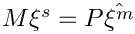Definition at line 1498 of file iterative_linear_solver.h.

## ◆ clean_up_memory()

template<typename MATRIX >
 void oomph::GMRES< MATRIX >::clean_up_memory ( )
inlineprivatevirtual

Cleanup data that's stored for resolve (if any has been stored)

Reimplemented from oomph::LinearSolver.

Definition at line 1357 of file iterative_linear_solver.h.

Referenced by oomph::GMRES< MATRIX >::disable_resolve(), and oomph::GMRES< MATRIX >::~GMRES().

## ◆ disable_iteration_restart()

template<typename MATRIX >
 void oomph::GMRES< MATRIX >::disable_iteration_restart ( )
inline

switches off iteration restart

Definition at line 1333 of file iterative_linear_solver.h.

References oomph::GMRES< MATRIX >::Iteration_restart.

## ◆ disable_resolve()

template<typename MATRIX >
 void oomph::GMRES< MATRIX >::disable_resolve ( )
inlinevirtual

Overload disable resolve so that it cleans up memory too.

Reimplemented from oomph::LinearSolver.

Definition at line 1254 of file iterative_linear_solver.h.

template<typename MATRIX >
 void oomph::GMRES< MATRIX >::enable_computation_of_gradient ( )
inlinevirtual

function to enable the computation of the gradient

Reimplemented from oomph::LinearSolver.

Definition at line 1261 of file iterative_linear_solver.h.

## ◆ enable_iteration_restart()

template<typename MATRIX >
 void oomph::GMRES< MATRIX >::enable_iteration_restart ( const unsigned & restart )
inline

switches on iteration restarting and takes as an argument the number of iterations after which the construction of the orthogonalisation basis vectors should be restarted

Definition at line 1326 of file iterative_linear_solver.h.

## ◆ generate_plane_rotation()

template<typename MATRIX >
 void oomph::GMRES< MATRIX >::generate_plane_rotation ( double & dx, double & dy, double & cs, double & sn )
inlineprivate

Helper function: Generate a plane rotation. This is done by finding the values of(i.e. cs) and \sin(\theta) (i.e. sn) such that: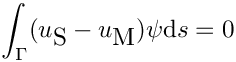where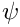. The values of a and b are given by: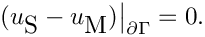andTaken from: Saad Y."Iterative methods for sparse linear systems", p.192

Definition at line 1453 of file iterative_linear_solver.h.

## ◆ iteration_restart()

template<typename MATRIX >
 bool oomph::GMRES< MATRIX >::iteration_restart ( ) const
inline

access function indicating whether restarted GMRES is used

Definition at line 1318 of file iterative_linear_solver.h.

References oomph::GMRES< MATRIX >::Iteration_restart.

## ◆ iterations()

template<typename MATRIX >
 unsigned oomph::GMRES< MATRIX >::iterations ( ) const
inlinevirtual

Number of iterations taken.

Implements oomph::IterativeLinearSolver.

Definition at line 1312 of file iterative_linear_solver.h.

References oomph::GMRES< MATRIX >::Iterations.

## ◆ operator=()

template<typename MATRIX >
 void oomph::GMRES< MATRIX >::operator= ( const GMRES< MATRIX > & )
delete

Broken assignment operator.

## ◆ resolve()

template<typename MATRIX >
 void oomph::GMRES< MATRIX >::resolve ( const DoubleVector & rhs, DoubleVector & result )
virtual

Re-solve the system defined by the last assembled Jacobian and the rhs vector specified here. Solution is returned in the vector result.

//////////////////////////////////////////////////////////////////// ////////////////////////////////////////////////////////////////////

\Short Re-solve the system defined by the last assembled Jacobian and the rhs vector specified here. Solution is returned in the vector result.

Reimplemented from oomph::LinearSolver.

Definition at line 2133 of file iterative_linear_solver.cc.

## ◆ set_preconditioner_LHS()

template<typename MATRIX >
 void oomph::GMRES< MATRIX >::set_preconditioner_LHS ( )
inline

Set left preconditioning (the default)

Definition at line 1339 of file iterative_linear_solver.h.

References oomph::GMRES< MATRIX >::Preconditioner_LHS.

## ◆ set_preconditioner_RHS()

template<typename MATRIX >
 void oomph::GMRES< MATRIX >::set_preconditioner_RHS ( )
inline

Enable right preconditioning.

Definition at line 1345 of file iterative_linear_solver.h.

References oomph::GMRES< MATRIX >::Preconditioner_LHS.

## ◆ solve() [1/3]

template<typename MATRIX >
 void oomph::GMRES< MATRIX >::solve ( DoubleMatrixBase *const & matrix_pt, const DoubleVector & rhs, DoubleVector & solution )
inlinevirtual

Linear-algebra-type solver: Takes pointer to a matrix and rhs vector and returns the solution of the linear system.

Reimplemented from oomph::LinearSolver.

Definition at line 1273 of file iterative_linear_solver.h.

## ◆ solve() [2/3]

template<typename MATRIX >
 void oomph::GMRES< MATRIX >::solve ( DoubleMatrixBase *const & matrix_pt, const Vector< double > & rhs, Vector< double > & result )
inlinevirtual

Linear-algebra-type solver: Takes pointer to a matrix and rhs vector and returns the solution of the linear system Call the broken base-class version. If you want this, please implement it.

Reimplemented from oomph::LinearSolver.

Definition at line 1299 of file iterative_linear_solver.h.

References oomph::LinearSolver::solve().

## ◆ solve() [3/3]

template<typename MATRIX >
 void oomph::GMRES< MATRIX >::solve ( Problem *const & problem_pt, DoubleVector & result )
virtual

Solver: Takes pointer to problem and returns the results vector which contains the solution of the linear system defined by the problem's fully assembled Jacobian and residual vector.

Implements oomph::LinearSolver.

Definition at line 2161 of file iterative_linear_solver.cc.

## ◆ solve_helper()

template<typename MATRIX >
 void oomph::GMRES< MATRIX >::solve_helper ( DoubleMatrixBase *const & matrix_pt, const DoubleVector & rhs, DoubleVector & solution )
private

General interface to solve function.

Linear-algebra-type solver: Takes pointer to a matrix and rhs vector and returns the solution of the linear system. based on the algorithm presented in Templates for the Solution of Linear Systems: Building Blocks for Iterative Methods, Barrett, Berry et al, SIAM, 2006 and the implementation in the IML++ library : http://math.nist.gov/iml++/.

Definition at line 2250 of file iterative_linear_solver.cc.

Referenced by oomph::GMRES< MATRIX >::solve().

## ◆ update()

template<typename MATRIX >
 void oomph::GMRES< MATRIX >::update ( const unsigned & k, const Vector< Vector< double > > & H, const Vector< double > & s, const Vector< DoubleVector > & v, DoubleVector & x )
inlineprivate

Helper function to update the result vector using the result, x=x_0+V_m*y.

Definition at line 1368 of file iterative_linear_solver.h.

## ◆ Iteration_restart

template<typename MATRIX >
 bool oomph::GMRES< MATRIX >::Iteration_restart
private

boolean indicating if iteration restarting is used

Definition at line 1518 of file iterative_linear_solver.h.

## ◆ Iterations

template<typename MATRIX >
 unsigned oomph::GMRES< MATRIX >::Iterations
private

Number of iterations taken.

Definition at line 1511 of file iterative_linear_solver.h.

Referenced by oomph::GMRES< MATRIX >::iterations().

## ◆ Matrix_can_be_deleted

template<typename MATRIX >
 bool oomph::GMRES< MATRIX >::Matrix_can_be_deleted
private

Boolean flag to indicate if the matrix pointed to be Matrix_pt can be deleted.

Definition at line 1529 of file iterative_linear_solver.h.

Referenced by oomph::GMRES< MATRIX >::clean_up_memory(), and oomph::GMRES< MATRIX >::solve().

## ◆ Matrix_pt

template<typename MATRIX >
 MATRIX* oomph::GMRES< MATRIX >::Matrix_pt
private

Pointer to matrix.

Definition at line 1521 of file iterative_linear_solver.h.

Referenced by oomph::GMRES< MATRIX >::clean_up_memory(), and oomph::GMRES< MATRIX >::solve().

## ◆ Preconditioner_application_time

template<typename MATRIX >
 double oomph::GMRES< MATRIX >::Preconditioner_application_time
private

Storage for the time spent applying the preconditioner.

Definition at line 1536 of file iterative_linear_solver.h.

Referenced by oomph::GMRES< MATRIX >::update().

## ◆ Preconditioner_LHS

template<typename MATRIX >
 bool oomph::GMRES< MATRIX >::Preconditioner_LHS
private

boolean indicating use of left hand preconditioning (if true) or right hand preconditioning (if false)

Definition at line 1533 of file iterative_linear_solver.h.

## ◆ Resolving

template<typename MATRIX >
 bool oomph::GMRES< MATRIX >::Resolving
private

Boolean flag to indicate if the solve is done in re-solve mode, bypassing setup of matrix and preconditioner.

Definition at line 1525 of file iterative_linear_solver.h.

Referenced by oomph::GMRES< MATRIX >::solve().

## ◆ Restart

template<typename MATRIX >
 unsigned oomph::GMRES< MATRIX >::Restart
private

The number of iterations before the iteration proceedure is restarted if iteration restart is used.

Definition at line 1515 of file iterative_linear_solver.h.

Referenced by oomph::GMRES< MATRIX >::enable_iteration_restart().

The documentation for this class was generated from the following files: